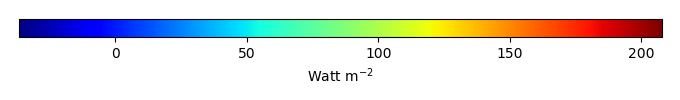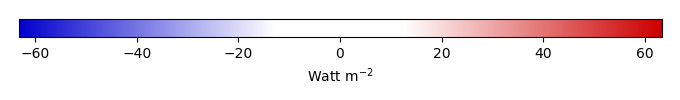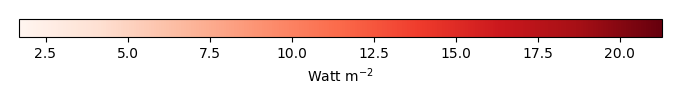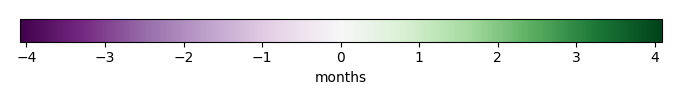# Mean State

Period Mean (original grids) [Watt m-2]
Model Period Mean (intersection) [Watt m-2]
Model Period Mean (complement) [Watt m-2]
Benchmark Period Mean (intersection) [Watt m-2]
Benchmark Period Mean (complement) [Watt m-2]
Bias [Watt m-2]
RMSE [Watt m-2]
Phase Shift [months]
Bias Score 
RMSE Score 
Seasonal Cycle Score 
Spatial Distribution Score 
Interannual Variability Score 
Overall Score 
Benchmark [-] 122.
CLM4 [-] 121. 121. 0.00 120. 164. 1.02 17.5 0.914 0.68 0.44 0.88 0.98 0.70 0.69
CLM4.5 [-] 117. 117. 0.00 120. 164. -2.83 18.4 0.892 0.66 0.44 0.88 0.93 0.70 0.68
CLM5 [-] 116. 116. 0.00 120. 164. -3.73 17.7 0.987 0.68 0.44 0.87 0.89 0.67 0.67
Period Mean (original grids) [Watt m-2]
Model Period Mean (intersection) [Watt m-2]
Model Period Mean (complement) [Watt m-2]
Benchmark Period Mean (intersection) [Watt m-2]
Benchmark Period Mean (complement) [Watt m-2]
Bias [Watt m-2]
RMSE [Watt m-2]
Phase Shift [months]
Bias Score 
RMSE Score 
Seasonal Cycle Score 
Spatial Distribution Score 
Interannual Variability Score 
Overall Score 
Benchmark [-] 102.
CLM4 [-] 103. 102. 0.00 101. 165. 1.84 19.4 0.981 0.74 0.50 0.86 0.98 0.71 0.72
CLM4.5 [-] 98.3 97.8 0.00 101. 165. -2.66 20.4 1.18 0.66 0.50 0.83 0.86 0.70 0.68
CLM5 [-] 97.7 97.2 0.00 101. 165. -3.30 19.8 1.28 0.67 0.51 0.81 0.89 0.65 0.67
Period Mean (original grids) [Watt m-2]
Model Period Mean (intersection) [Watt m-2]
Model Period Mean (complement) [Watt m-2]
Benchmark Period Mean (intersection) [Watt m-2]
Benchmark Period Mean (complement) [Watt m-2]
Bias [Watt m-2]
RMSE [Watt m-2]
Phase Shift [months]
Bias Score 
RMSE Score 
Seasonal Cycle Score 
Spatial Distribution Score 
Interannual Variability Score 
Overall Score 
Benchmark [-] 112.
CLM4 [-] 109. 109. 0.00 110. 159. 0.387 20.4 0.939 0.74 0.57 0.87 0.92 0.65 0.72
CLM4.5 [-] 104. 104. 0.00 110. 159. -4.74 21.3 0.911 0.68 0.57 0.87 0.85 0.66 0.70
CLM5 [-] 105. 105. 0.00 110. 159. -3.73 20.8 1.22 0.71 0.57 0.81 0.87 0.61 0.69
Period Mean (original grids) [Watt m-2]
Model Period Mean (intersection) [Watt m-2]
Model Period Mean (complement) [Watt m-2]
Benchmark Period Mean (intersection) [Watt m-2]
Benchmark Period Mean (complement) [Watt m-2]
Bias [Watt m-2]
RMSE [Watt m-2]
Phase Shift [months]
Bias Score 
RMSE Score 
Seasonal Cycle Score 
Spatial Distribution Score 
Interannual Variability Score 
Overall Score 
Benchmark [-] 43.3
CLM4 [-] 28.6 28.8 0.00 43.2 44.3 -15.2 27.1 0.307 0.76 0.67 0.98 0.89 0.77 0.79
CLM4.5 [-] 33.7 33.7 0.00 43.2 44.3 -10.9 23.1 0.171 0.81 0.70 0.99 0.79 0.73 0.79
CLM5 [-] 33.3 33.3 0.00 43.2 44.3 -11.6 23.4 0.172 0.81 0.70 0.99 0.78 0.76 0.79
Period Mean (original grids) [Watt m-2]
Model Period Mean (intersection) [Watt m-2]
Model Period Mean (complement) [Watt m-2]
Benchmark Period Mean (intersection) [Watt m-2]
Benchmark Period Mean (complement) [Watt m-2]
Bias [Watt m-2]
RMSE [Watt m-2]
Phase Shift [months]
Bias Score 
RMSE Score 
Seasonal Cycle Score 
Spatial Distribution Score 
Interannual Variability Score 
Overall Score 
Benchmark [-] 144.
CLM4 [-] 135. 134. 0.00 140. 160. -1.65 19.9 1.09 0.62 0.42 0.84 0.80 0.64 0.62
CLM4.5 [-] 132. 132. 0.00 140. 160. -3.26 19.9 1.13 0.63 0.42 0.84 0.87 0.63 0.64
CLM5 [-] 128. 127. 0.00 140. 160. -7.88 20.6 0.968 0.60 0.43 0.86 0.90 0.60 0.64
Period Mean (original grids) [Watt m-2]
Model Period Mean (intersection) [Watt m-2]
Model Period Mean (complement) [Watt m-2]
Benchmark Period Mean (intersection) [Watt m-2]
Benchmark Period Mean (complement) [Watt m-2]
Bias [Watt m-2]
RMSE [Watt m-2]
Phase Shift [months]
Bias Score 
RMSE Score 
Seasonal Cycle Score 
Spatial Distribution Score 
Interannual Variability Score 
Overall Score 
Benchmark [-] 62.5
CLM4 [-] 53.1 53.1 0.00 62.3 75.7 -8.90 19.5 0.0965 0.85 0.75 0.99 0.84 0.63 0.80
CLM4.5 [-] 50.6 50.6 0.00 62.3 75.7 -11.4 21.7 0.0904 0.82 0.73 0.99 0.86 0.64 0.79
CLM5 [-] 48.1 48.1 0.00 62.3 75.7 -13.9 23.3 0.107 0.79 0.72 0.99 0.94 0.67 0.80
Period Mean (original grids) [Watt m-2]
Model Period Mean (intersection) [Watt m-2]
Model Period Mean (complement) [Watt m-2]
Benchmark Period Mean (intersection) [Watt m-2]
Benchmark Period Mean (complement) [Watt m-2]
Bias [Watt m-2]
RMSE [Watt m-2]
Phase Shift [months]
Bias Score 
RMSE Score 
Seasonal Cycle Score 
Spatial Distribution Score 
Interannual Variability Score 
Overall Score 
Benchmark [-] 72.7
CLM4 [-] 65.4 65.4 0.00 72.1 94.9 -5.48 20.7 0.403 0.80 0.66 0.97 0.97 0.62 0.78
CLM4.5 [-] 64.1 64.0 0.00 72.1 94.9 -6.72 21.3 0.404 0.79 0.66 0.97 0.98 0.62 0.78
CLM5 [-] 62.7 62.6 0.00 72.1 94.9 -8.19 21.7 0.393 0.79 0.65 0.97 0.99 0.63 0.78
Period Mean (original grids) [Watt m-2]
Model Period Mean (intersection) [Watt m-2]
Model Period Mean (complement) [Watt m-2]
Benchmark Period Mean (intersection) [Watt m-2]
Benchmark Period Mean (complement) [Watt m-2]
Bias [Watt m-2]
RMSE [Watt m-2]
Phase Shift [months]
Bias Score 
RMSE Score 
Seasonal Cycle Score 
Spatial Distribution Score 
Interannual Variability Score 
Overall Score 
Benchmark [-] 109.
CLM4 [-] 99.8 99.4 0.00 105. 157. -1.87 23.2 0.448 0.72 0.53 0.96 0.98 0.62 0.72
CLM4.5 [-] 95.6 95.3 0.00 105. 157. -5.50 23.3 0.470 0.71 0.53 0.95 0.98 0.60 0.72
CLM5 [-] 94.4 94.1 0.00 105. 157. -6.92 23.7 0.544 0.72 0.53 0.95 0.96 0.56 0.71
Period Mean (original grids) [Watt m-2]
Model Period Mean (intersection) [Watt m-2]
Model Period Mean (complement) [Watt m-2]
Benchmark Period Mean (intersection) [Watt m-2]
Benchmark Period Mean (complement) [Watt m-2]
Bias [Watt m-2]
RMSE [Watt m-2]
Phase Shift [months]
Bias Score 
RMSE Score 
Seasonal Cycle Score 
Spatial Distribution Score 
Interannual Variability Score 
Overall Score 
Benchmark [-] 41.8
CLM4 [-] 21.4 21.7 0.00 41.9 41.5 -20.7 27.1 0.255 0.68 0.68 0.98 0.95 0.72 0.78
CLM4.5 [-] 24.5 24.9 0.00 41.9 41.5 -17.9 24.3 0.292 0.72 0.69 0.98 0.93 0.72 0.79
CLM5 [-] 25.1 25.4 0.00 41.9 41.5 -17.5 23.3 0.253 0.72 0.70 0.98 0.94 0.75 0.80
Period Mean (original grids) [Watt m-2]
Model Period Mean (intersection) [Watt m-2]
Model Period Mean (complement) [Watt m-2]
Benchmark Period Mean (intersection) [Watt m-2]
Benchmark Period Mean (complement) [Watt m-2]
Bias [Watt m-2]
RMSE [Watt m-2]
Phase Shift [months]
Bias Score 
RMSE Score 
Seasonal Cycle Score 
Spatial Distribution Score 
Interannual Variability Score 
Overall Score 
Benchmark [-] 96.5
CLM4 [-] 93.7 93.7 0.00 93.2 138. 2.21 19.9 0.329 0.78 0.67 0.98 0.86 0.64 0.77
CLM4.5 [-] 93.0 93.0 0.00 93.2 138. 1.62 19.9 0.339 0.78 0.67 0.97 0.84 0.65 0.76
CLM5 [-] 92.5 92.4 0.00 93.2 138. 1.15 20.2 0.330 0.78 0.67 0.97 0.87 0.64 0.76
Period Mean (original grids) [Watt m-2]
Model Period Mean (intersection) [Watt m-2]
Model Period Mean (complement) [Watt m-2]
Benchmark Period Mean (intersection) [Watt m-2]
Benchmark Period Mean (complement) [Watt m-2]
Bias [Watt m-2]
RMSE [Watt m-2]
Phase Shift [months]
Bias Score 
RMSE Score 
Seasonal Cycle Score 
Spatial Distribution Score 
Interannual Variability Score 
Overall Score 
Benchmark [-] 64.4
CLM4 [-] 56.1 56.2 0.00 61.9 90.5 -3.80 19.2 0.270 0.86 0.74 0.98 0.96 0.65 0.82
CLM4.5 [-] 57.7 57.7 0.00 61.9 90.5 -2.31 18.2 0.284 0.87 0.75 0.98 0.98 0.65 0.83
CLM5 [-] 54.4 54.4 0.00 61.9 90.5 -5.49 19.0 0.289 0.86 0.74 0.98 0.98 0.64 0.82
Period Mean (original grids) [Watt m-2]
Model Period Mean (intersection) [Watt m-2]
Model Period Mean (complement) [Watt m-2]
Benchmark Period Mean (intersection) [Watt m-2]
Benchmark Period Mean (complement) [Watt m-2]
Bias [Watt m-2]
RMSE [Watt m-2]
Phase Shift [months]
Bias Score 
RMSE Score 
Seasonal Cycle Score 
Spatial Distribution Score 
Interannual Variability Score 
Overall Score 
Benchmark [-] 115.
CLM4 [-] 68.2 68.2 0.00 77.4 133. -7.29 22.9 0.534 0.71 0.60 0.94 0.93 0.63 0.73
CLM4.5 [-] 67.2 67.2 0.00 77.4 133. -8.11 22.8 0.548 0.70 0.60 0.94 0.94 0.63 0.73
CLM5 [-] 65.7 65.7 0.00 77.4 133. -10.1 23.7 0.563 0.68 0.60 0.93 0.92 0.62 0.73
Period Mean (original grids) [Watt m-2]
Model Period Mean (intersection) [Watt m-2]
Model Period Mean (complement) [Watt m-2]
Benchmark Period Mean (intersection) [Watt m-2]
Benchmark Period Mean (complement) [Watt m-2]
Bias [Watt m-2]
RMSE [Watt m-2]
Phase Shift [months]
Bias Score 
RMSE Score 
Seasonal Cycle Score 
Spatial Distribution Score 
Interannual Variability Score 
Overall Score 
Benchmark [-] 54.9
CLM4 [-] 54.1 54.2 0.00 51.3 143. 4.96 25.9 0.956 0.66 0.52 0.88 0.83 0.54 0.66
CLM4.5 [-] 54.2 54.2 0.00 51.3 143. 5.12 25.9 0.973 0.66 0.52 0.88 0.84 0.55 0.66
CLM5 [-] 54.2 54.2 0.00 51.3 143. 5.15 26.1 0.880 0.66 0.52 0.89 0.85 0.55 0.67
Period Mean (original grids) [Watt m-2]
Model Period Mean (intersection) [Watt m-2]
Model Period Mean (complement) [Watt m-2]
Benchmark Period Mean (intersection) [Watt m-2]
Benchmark Period Mean (complement) [Watt m-2]
Bias [Watt m-2]
RMSE [Watt m-2]
Phase Shift [months]
Bias Score 
RMSE Score 
Seasonal Cycle Score 
Spatial Distribution Score 
Interannual Variability Score 
Overall Score 
Benchmark [-] 44.9
CLM4 [-] 27.6 27.5 0.00 44.8 45.6 -18.7 25.4 0.128 0.72 0.71 0.99 0.93 0.76 0.80
CLM4.5 [-] 31.0 30.9 0.00 44.8 45.6 -15.8 22.6 0.129 0.75 0.73 0.99 0.92 0.75 0.81
CLM5 [-] 28.4 28.3 0.00 44.8 45.6 -18.6 24.6 0.122 0.72 0.72 0.99 0.87 0.78 0.80
Period Mean (original grids) [Watt m-2]
Model Period Mean (intersection) [Watt m-2]
Model Period Mean (complement) [Watt m-2]
Benchmark Period Mean (intersection) [Watt m-2]
Benchmark Period Mean (complement) [Watt m-2]
Bias [Watt m-2]
RMSE [Watt m-2]
Phase Shift [months]
Bias Score 
RMSE Score 
Seasonal Cycle Score 
Spatial Distribution Score 
Interannual Variability Score 
Overall Score 
Benchmark [-] 134.
CLM4 [-] 108. 109. 0.00 127. 169. -13.1 24.2 0.706 0.64 0.60 0.92 0.95 0.66 0.73
CLM4.5 [-] 108. 109. 0.00 127. 169. -12.9 24.1 0.704 0.64 0.60 0.92 0.96 0.67 0.73
CLM5 [-] 107. 108. 0.00 127. 169. -14.0 24.1 0.799 0.64 0.60 0.90 0.95 0.62 0.72
Period Mean (original grids) [Watt m-2]
Model Period Mean (intersection) [Watt m-2]
Model Period Mean (complement) [Watt m-2]
Benchmark Period Mean (intersection) [Watt m-2]
Benchmark Period Mean (complement) [Watt m-2]
Bias [Watt m-2]
RMSE [Watt m-2]
Phase Shift [months]
Bias Score 
RMSE Score 
Seasonal Cycle Score 
Spatial Distribution Score 
Interannual Variability Score 
Overall Score 
Benchmark [-] 99.6
CLM4 [-] 89.8 90.0 0.00 97.8 136. -6.24 17.7 0.276 0.88 0.72 0.98 0.99 0.65 0.82
CLM4.5 [-] 87.1 87.2 0.00 97.8 136. -8.79 18.8 0.305 0.83 0.72 0.98 0.99 0.65 0.82
CLM5 [-] 82.0 82.1 0.00 97.8 136. -14.0 21.5 0.352 0.75 0.72 0.97 1.0 0.62 0.80
Period Mean (original grids) [Watt m-2]
Model Period Mean (intersection) [Watt m-2]
Model Period Mean (complement) [Watt m-2]
Benchmark Period Mean (intersection) [Watt m-2]
Benchmark Period Mean (complement) [Watt m-2]
Bias [Watt m-2]
RMSE [Watt m-2]
Phase Shift [months]
Bias Score 
RMSE Score 
Seasonal Cycle Score 
Spatial Distribution Score 
Interannual Variability Score 
Overall Score 
Benchmark [-] 72.2
CLM4 [-] 61.8 61.9 0.00 72.0 77.2 -10.0 18.3 0.102 0.84 0.77 0.99 0.97 0.65 0.83
CLM4.5 [-] 62.4 62.5 0.00 72.0 77.2 -9.43 18.0 0.108 0.84 0.77 0.99 0.99 0.64 0.84
CLM5 [-] 58.6 58.7 0.00 72.0 77.2 -13.2 20.6 0.102 0.79 0.76 0.99 1.0 0.67 0.83
Period Mean (original grids) [Watt m-2]
Model Period Mean (intersection) [Watt m-2]
Model Period Mean (complement) [Watt m-2]
Benchmark Period Mean (intersection) [Watt m-2]
Benchmark Period Mean (complement) [Watt m-2]
Bias [Watt m-2]
RMSE [Watt m-2]
Phase Shift [months]
Bias Score 
RMSE Score 
Seasonal Cycle Score 
Spatial Distribution Score 
Interannual Variability Score 
Overall Score 
Benchmark [-] 81.6
CLM4 [-] 73.7 73.9 0.00 81.1 116. -6.54 19.2 0.310 0.81 0.72 0.98 0.99 0.68 0.81
CLM4.5 [-] 72.2 72.5 0.00 81.1 116. -7.93 20.1 0.296 0.79 0.71 0.98 0.99 0.68 0.81
CLM5 [-] 72.0 72.2 0.00 81.1 116. -8.22 20.4 0.314 0.79 0.71 0.98 0.99 0.66 0.81
Period Mean (original grids) [Watt m-2]
Model Period Mean (intersection) [Watt m-2]
Model Period Mean (complement) [Watt m-2]
Benchmark Period Mean (intersection) [Watt m-2]
Benchmark Period Mean (complement) [Watt m-2]
Bias [Watt m-2]
RMSE [Watt m-2]
Phase Shift [months]
Bias Score 
RMSE Score 
Seasonal Cycle Score 
Spatial Distribution Score 
Interannual Variability Score 
Overall Score 
Benchmark [-] 132.
CLM4 [-] 127. 127. 0.00 131. 178. -3.71 19.3 0.888 0.72 0.39 0.87 0.91 0.63 0.65
CLM4.5 [-] 124. 124. 0.00 131. 178. -6.16 20.1 0.926 0.66 0.39 0.87 0.96 0.62 0.65
CLM5 [-] 122. 122. 0.00 131. 178. -7.96 20.3 0.991 0.62 0.39 0.86 0.95 0.60 0.64
Period Mean (original grids) [Watt m-2]
Model Period Mean (intersection) [Watt m-2]
Model Period Mean (complement) [Watt m-2]
Benchmark Period Mean (intersection) [Watt m-2]
Benchmark Period Mean (complement) [Watt m-2]
Bias [Watt m-2]
RMSE [Watt m-2]
Phase Shift [months]
Bias Score 
RMSE Score 
Seasonal Cycle Score 
Spatial Distribution Score 
Interannual Variability Score 
Overall Score 
Benchmark [-] 117.
CLM4 [-] 114. 114. 0.00 117. 145. -2.62 19.8 0.957 0.78 0.55 0.87 0.95 0.66 0.73
CLM4.5 [-] 111. 111. 0.00 117. 145. -5.30 20.3 0.967 0.74 0.55 0.87 0.94 0.67 0.72
CLM5 [-] 108. 108. 0.00 117. 145. -8.04 20.3 0.874 0.73 0.56 0.88 0.97 0.62 0.72
Period Mean (original grids) [Watt m-2]
Model Period Mean (intersection) [Watt m-2]
Model Period Mean (complement) [Watt m-2]
Benchmark Period Mean (intersection) [Watt m-2]
Benchmark Period Mean (complement) [Watt m-2]
Bias [Watt m-2]
RMSE [Watt m-2]
Phase Shift [months]
Bias Score 
RMSE Score 
Seasonal Cycle Score 
Spatial Distribution Score 
Interannual Variability Score 
Overall Score 
Benchmark [-] 95.7
CLM4 [-] 91.7 91.1 0.00 92.5 124. -2.48 25.1 0.409 0.74 0.68 0.96 0.99 0.57 0.77
CLM4.5 [-] 90.4 89.8 0.00 92.5 124. -3.56 25.1 0.410 0.74 0.68 0.96 0.99 0.57 0.77
CLM5 [-] 90.8 90.1 0.00 92.5 124. -3.40 24.9 0.391 0.74 0.68 0.96 0.99 0.57 0.77

# Temporally integrated period mean

BENCHMARK MEANMODEL MEANBIASBIAS SCORERMSERMSE SCOREBENCHMARK INTERANNUAL VARIABILITYMODEL INTERANNUAL VARIABILITYINTERANNUAL VARIABILITY SCOREBENCHMARK MAX MONTHMODEL MAX MONTHDIFFERENCE IN MAX MONTHSEASONAL CYCLE SCORESPATIAL TAYLOR DIAGRAMMODEL COLORS# Spatially integrated regional mean

MODEL COLORSREGIONAL MEANANNUAL CYCLEMONTHLY ANOMALYANNUAL CYCLE# All Models

BenchmarkCLM4CLM4.5CLM5# Data Information

creation_date: Tue Jul 1 08:16:58 PDT 2014

source_file: This product is generated from monthly 1 degree GEWEX SRB Radiation observations